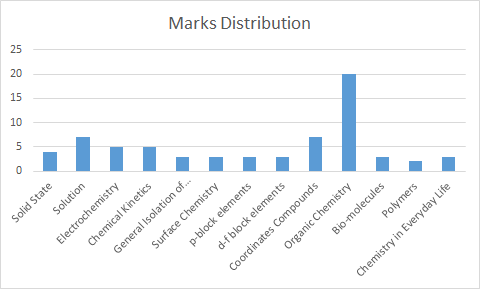# CBSE Class 12 Chemistry Post Paper Analysis 2019

The Central Board of Secondary Education has conducted the Chemistry paper of class 12 today i.e 12th March 2019 across various exam centers in India. Students were given 15 minutes for only reading the chemistry paper and they started writing the answers from 10:30 am. Chemistry is one of the important subjects for CBSE science stream students. Therefore we are providing the CBSE Class 12 Chemistry Paper Analysis 2019. With the help of this CBSE Class 12 Chemistry 2019 Exam review students can evaluate their performance.

Students as well as teachers can find the paper analysis of 2019 CBSE Class 10th & 12th Board Exams along with the previous years paper analysis by clicking on the link below:

### CBSE Class 12 Chemistry Paper 2019 Specifics:

The CBSE Class 12 Chemistry paper is of total 100 marks. The 70 marks is for the Chemistry theory paper & 30 marks for practicals.

 Exam Date of CBSE Class 12 Chemistry Paper 12th March 2019 Exam Timing 10:30 am – 1:30 pm Exam Duration 3 Hrs Chemistry Paper Marks Distribution 70 Marks (Theory Paper) 30 Marks (Practical Exams)

## CBSE Class 12 Chemistry Exam Analysis 2019

The Chemistry paper of CBSE Class 12 consist of total 27 questions which were divided into 4 sections as mentioned below:

1. Section A: This section consists of very short answer questions of 1 mark. Under this section there were total 5 Qs ranging from questions number from 1 to 5.
2. Section B: This section consists of short answer questions of 2 marks. Under this section there were total 7 Qs ranging from questions number from 6 to 12.
3. Section C: This section also consists of short answer questions of 3 marks. Under this section there were total 12 Qs ranging from questions number from 13 to 24.
4. Section D: This section consists of long answer questions of 5 marks. Under this section there were total 3 Qs ranging from questions number from 25 to 27.

The questions asked in the exam were direct & nothing came out of the CBSE Class 12 syllabus.

 Section Question Type No. of Questions Total Marks Section A Very short answer Questions Consisting of 1 Marks 5 5 Section B Short Answers Consisting of 2 Marks 7 14 Section C Short Answers Consisting of 3 Marks 12 36 Section D Long Answers Consisting of 5 Marks 3 15 Total 27 70

### CBSE Class 12 Chemistry Paper Review 2019

Compared to the last year CBSE Class 12 Chemistry paper, this year the paper was easier. As the CBSE Class 12 Chemistry 2019 paper was not lengthy therefore some of the students completed it before the allotted time. Most of the questions were asked from the organic chemistry & also there were few numericals asked in the CBSE Chemistry 12th paper. Moreover, there were some tricky questions from the P BLOCK element chapter. However the overall paper was of moderate level.

### Chapterwise Weightage of the CBSE Class 12 Chemistry Paper

Students can see topic wise marks distribution of the Class 12 CBSE Chemistry paper below:

 Chapters Marks Distribution Solid State 4 Solution 7 Electrochemistry 5 Chemical Kinetics 5 General Isolation of Elements 3 Surface Chemistry 3 p-block elements 3 d-f block elements 3 Coordinates Compounds 7 Organic Chemistry 20 Biomolecules 3 Polymers 2 Chemistry in Everyday Life 3 Total 70The chemistry paper is over. As per the CBSE Class 12 Date Sheet, the next difficult paper for students will be of mathematics on 18th March 2019. For mathematics paper, students should solve the CBSE 12th Previous Year Question Papers. Moreover, we have also provided the links to important study material that will help in CBSE 12th board exam preparation.

Important Links to Refer:

Stay tuned for the latest update on CBSE Board Exam. Download BYJU’S App for interactive Maths & Science Videos.

All the Best for Next Paper!

 NCERT Related Articles NCERT Solutions for Class 12 NCERT Solutions for Class 11 NCERT Solutions for Class 10 NCERT Solutions for Class 12 Maths NCERT Solutions for Class 11 Maths NCERT Solutions for Class 10 Maths NCERT Solutions for Class 12 Physics NCERT Solutions for Class 11 Physics NCERT Solutions for Class 10 Science NCERT Solutions for Class 12 Chemistry NCERT Solutions for Class 11 Chemistry NCERT Solutions for Class 6 NCERT Solutions for Class 12 Biology NCERT Solutions for Class 11 Biology NCERT Solutions for Class 6 Maths NCERT Solutions for Class 9 NCERT Solutions for Class 8 NCERT Solutions for Class 6 Science NCERT Solutions for Class 9 Maths NCERT Solutions for Class 8 Maths NCERT Solutions for Class 4 NCERT Solutions for Class 9 Science NCERT Solutions for Class 8 Science NCERT Solutions for Class 4 Maths NCERT Solutions for Class 5 NCERT Solutions for Class 7 NCERT Solutions for Class 7 Maths NCERT Solutions for Class 4 Science NCERT Solutions for Class 5 Maths NCERT Solutions for Class 7 Science NCERT Solutions NCERT Books NCERT Solutions for Class 5 Science